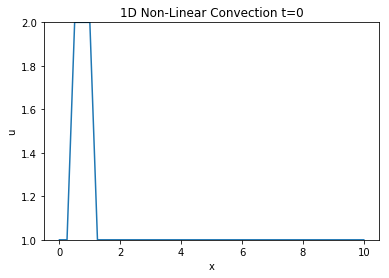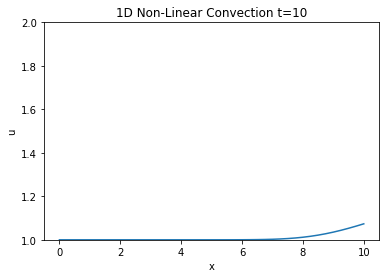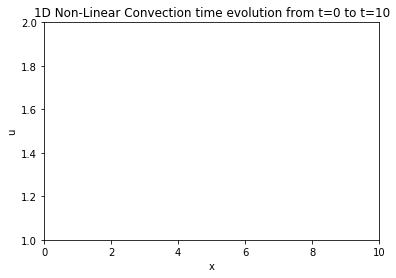# Step 2: Non-linear Convection¶

I began this trek along the 12 steps of Navier stokes with the extremely simple 1-D linear convection equation shown below:

$$\frac{\partial u}{\partial t} + c \frac{\partial u}{\partial x} = 0$$

Next, we up the ante and present a new more complex model known as the 1D convection equation (notice the lack of the word linear) :

$$\frac{\partial u}{\partial t} + u \frac{\partial u}{\partial x} = 0$$

Now, instead of a constant factor c multiplying we have the solution u doing that instead. Thus this makes our convection equation behave nonlinearly. We shall follow the exact discretization steps outline in Step 1 with the following result:

$$\frac{u_i^{n+1} - u^n_i}{\Delta t} + u^n_i \frac{u_{i}^{n} - u^n_{i-1}}{\Delta t} = 0$$

Solving for the same unknown value $u_i^{n+1}$:

$$u_i^{n+1} = u^n_i - u^n_i \frac{\Delta t}{\Delta x}(u_i^n - u^n_{i-1})$$

The following step is a condensed version of what we did to set up initial conditions, variables and libraries and is taken directly from the previous notebook.

## Libraries & Initial Conditions¶

In :
# Adding inline command to make plots appear under comments
import numpy as np
import matplotlib.pyplot as plt
import time, sys
%matplotlib inline

#Same initial conditions as in step 1
grid_length = 10
grid_points = 41
dx = grid_length / (grid_points - 1)
nt = 400
dt = 0.025

#Initiallizing the shape of the wave to the same one from step 1 and displaying it
u = np.ones(grid_points)
u[int(.5/ dx):int(1 / dx + 1)] = 2
plt.plot(np.linspace(0,grid_length,grid_points), u);
plt.ylim(1,2);
plt.xlabel('x')
plt.ylabel('u')
plt.title('1D Non-Linear Convection t=0');## Applying the discretization¶

Now we apply the discretization as outlined above and check out the final results.

In :
un = np.ones(grid_points)

for n in range(nt): #Runs however many timesteps you set earlier
un = u.copy()   #copy the u array to not overwrite values
for i in range(1,grid_points):
u[i] = un[i] - un[i] * dt/dx * (un[i]-un[i-1])

In :
plt.plot(np.linspace(0,grid_length,grid_points), u);
plt.ylim(1,2);
plt.xlabel('x')
plt.ylabel('u')
plt.title('1D Non-Linear Convection t=10');### Results¶

Looks pretty unchanged, to have a better idea if it behaved the same way we will take a look at the animation.

## Animating the wave moving¶

In :
#Imports for animation and display within a jupyter notebook
from matplotlib import animation, rc
from IPython.display import HTML

#Generating the figure that will contain the animation
fig, ax = plt.subplots()
ax.set_xlim(( 0, grid_length))
ax.set_ylim((1, 2))
line, = ax.plot([], [], lw=2)
plt.xlabel('x')
plt.ylabel('u')
plt.title('1D Non-Linear Convection time evolution from t=0 to t=10');
#Resetting the U wave back to initial conditions
u = np.ones(grid_points)
u[int(.5/ dx):int(1 / dx + 1)] = 2In :
#Initialization function for funcanimation
def init():
line.set_data([], [])
return (line,)

In :
#Main animation function, each frame represents a time step in our calculation
def animate(j):
x = np.linspace(0, grid_length, grid_points)
un = u.copy()   #copy the u array to not overwrite values
for i in range(1,grid_points):
u[i] = un[i] - un[i] * dt/dx * (un[i]-un[i-1])
line.set_data(x, u)
return (line,)

In :
anim = animation.FuncAnimation(fig, animate, init_func=init,
frames=nt, interval=20)
HTML(anim.to_jshtml())

Out:

Once Loop Reflect